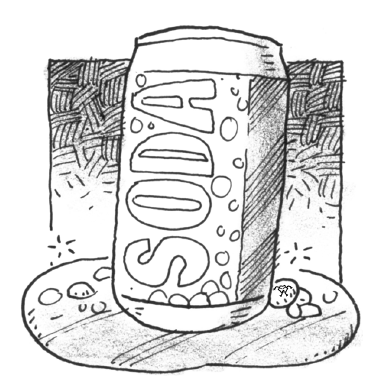### Home > APCALC > Chapter 9 > Lesson 9.1.2 > Problem9-26

9-26.

If a hole is punched in the bottom of a tall open can, the rate at which liquid leaks out is roughly proportional to the square root of the depth of the remaining liquid. Unfortunately, Jose’s pesky little brother just used a pen to punch a hole in the bottom of his $16$ cm tall (completely full) can of soda, decreasing the depth of the precious liquid at the rate of $12$ cm/min. What is the height of the soda in the can after $20$ seconds?$\frac{dh}{dt} = k\sqrt{h}$

$\text{When }h=16\text{, }\frac{dh}{dt}=-12\text{. Use this information to solve for }k{.}$